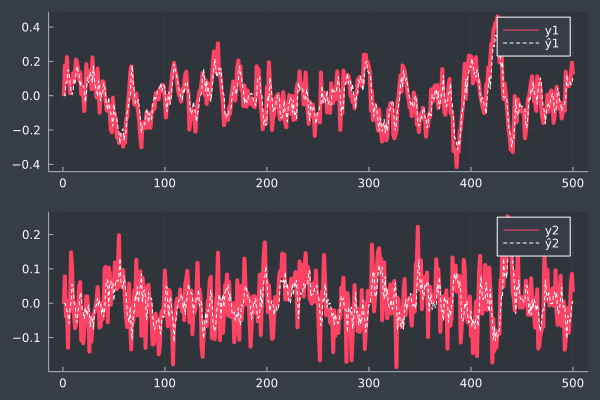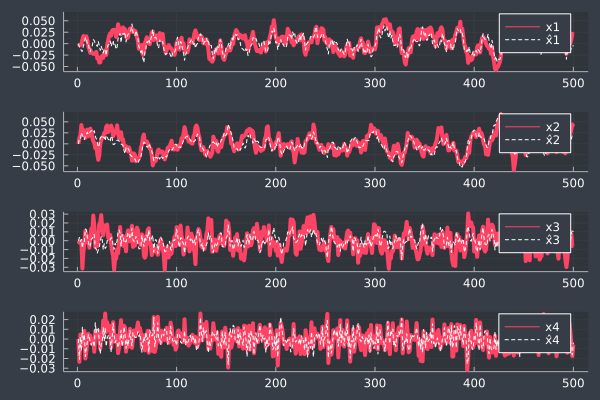# Comparing the basic and extended Kalman filters

This notebook doesn't offer much in the way of explanation, but explores implementations of the basic and extended Kalman filters and compares them for different nonlinearities. This was made with Pluto.jl, the reactive notebook for Julia.

using LinearAlgebra, PlotThemes, Plots
begin
theme(:dark)
gr()
end
Plots.GRBackend()

## System setup

nx = size(A, 1)
4
Q = 1e-2*I(nx)
4×4 Diagonal{Float64, Vector{Float64}}:
0.01   ⋅     ⋅     ⋅
⋅    0.01   ⋅     ⋅
⋅     ⋅    0.01   ⋅
⋅     ⋅     ⋅    0.01
R = 1e-3*rand(ny, ny)
2×2 Matrix{Float64}:
0.000148677  0.000727344
7.94516e-5   0.000904293
ny = 2
2
C = 5*(2*rand(ny, nx) .- 1)
2×4 Matrix{Float64}:
-3.83543   4.42741   -0.925343  -4.37376
2.41531  -0.441565   3.63074   -4.21982
A = Tridiagonal([-.1, 0, 0], [.9, .9, .6, .01], [0.1, 0, 0])
4×4 Tridiagonal{Float64, Vector{Float64}}:
0.9  0.1   ⋅    ⋅
-0.1  0.9  0.0   ⋅
⋅   0.0  0.6  0.0
⋅    ⋅   0.0  0.01
function meas(x)
C*x + R*randn(ny)
end
meas (generic function with 1 method)
function sim(T)
X = zeros(nx, T)
Y = zeros(ny, T)
Y[:, 1] = meas(X[:, 1])
for t in 2:T
X[:, t] = A*X[:, t-1] + Q*randn(nx)
Y[:, t] = meas(X[:, t])
end
return X, Y
end
sim (generic function with 1 method)
X, Y = sim(500)
([0.0 -0.00304089532961092 … 0.021776565996900765 0.020161270145038598; 0.0 0.013148729172110036 … 0.043828185113484644 0.03892005610669178; 0.0 -0.002263357615255907 … -0.005985980156374349 -0.0057379214802191405; 0.0 -0.02347516203690685 … -0.017582460136818145 -0.005736983094668349], [-0.001224111468893593 0.17509465540588978 … 0.19266990282332822 0.12392340218887515; -0.0014190368765992151 0.07833241652533991 … 0.08526559564733202 0.0331403889946617])

## Kalman filter

We'll return the predicted x trajectories $\hat{X}$ as well as the one-step-ahead output predictions $\hat{Y}$

function kf(Y)
T = size(Y, 2)
X̂ = zeros(nx, T)
P = zeros(nx, nx)
Ŷ = zeros(size(Y))
Ŷ[:, 1] = C*X̂[:, 1]
for t in 2:T
# predict
x̂₊ = A*X̂[:, t-1]
Ŷ[:, t] = C*x̂₊
P₊ = A*P*A' + Q
# update
K = P₊*C'*inv(C*P₊*C' + R)
X̂[:, t] = x̂₊ + K*(Y[:, t] - C*x̂₊)
P = (I - K*C)*P₊*(I - K*C)' + K*R*K'
# Ŷ[:, t] = C*X̂[:, t]
end
return X̂, Ŷ
end
kf (generic function with 1 method)
X̂, Ŷ = kf(Y)
([0.0 -0.007550090867988593 … -0.008219272435918894 -0.0035408422295270365; 0.0 0.012908496004416981 … 0.022709330945614732 0.023316376410793455; 0.0 0.0036250333583013146 … 0.012523457920984908 0.009973561966994776; 0.0 -0.021072065435554066 … -0.016467377387537237 -0.003724922391327674], [0.0 0.0 … 0.06658760545193752 0.10755724453634861; 0.0 0.0 … -0.003618258053732906 0.006206891556976631])
begin
yplots = []
for i in 1:ny
p = plot(Y[i, :], lw=4, label="y$i") plot!(Ŷ[i, :], label="ŷ$i", c=:white, linestyle=:dash)
push!(yplots, p)
end
plot(yplots..., layout=(ny, 1), link=:both, legend=true)
endbegin
xplots = []
for i in 1:nx
p = plot(X[i, :], lw=4, label="x$i") plot!(X̂[i, :], label="x̂$i", c=:white, linestyle=:dash)
push!(xplots, p)
end
plot(xplots..., layout=(nx, 1), link=:both)
end## Extended Kalman Filter

Let's set up a nonlinear system and see how the standard Kalman filter does.

Here, the nonlinearities are contained in $f$ and $h$:

$$x_{t+1} = f(x_t) + w \quad y_t = h(x_t) + v$$

function nlsim(T, f, h)
X = zeros(nx, T)
Y = zeros(ny, T)
Y[:, 1] = meas(X[:, 1])
for t in 2:T
X[:, t] = f(X[:, t-1]) + Q*randn(nx)
Y[:, t] = h(X[:, t]) + R*randn(ny)
end
return X, Y
end

nlsim (generic function with 1 method)
expneg(x) = exp(-x)
expneg (generic function with 1 method)
σ(x) = 1 / (1 + exp(-x))
σ (generic function with 1 method)
x²(x) = x^2
x² (generic function with 1 method)
relu(x) = x > 0 ? x : 0
relu (generic function with 1 method)
dampsin(x) = sin(x)*exp(-x^2)
dampsin (generic function with 1 method)
nonlinearities = [cos, expneg, relu, σ, atan, x², dampsin]
7-element Vector{Function}:
cos (generic function with 17 methods)
expneg (generic function with 1 method)
relu (generic function with 1 method)
σ (generic function with 1 method)
atan (generic function with 35 methods)
x² (generic function with 1 method)
dampsin (generic function with 1 method)
using Printf: @sprintf
function test_filt_nonlin(nlfunc, filt)
ps = []
for nl in nonlinearities
f(x) = nlfunc in (:both, :f) ? nl.(A*x) : A*x
h(x) = nlfunc in (:both, :h) ? nl.(C*x) : C*x

X, Y = nlsim(100, f, h)
pX = plot(X', lw=4, label="x")
pY = plot(Y', lw=4, label="y")

if filt == kf
X̂, Ŷ = filt(Y)
elseif filt == ekf
X̂, Ŷ = filt(Y, f, h)
end
X̃norm = @sprintf("%.2f", norm(X̂-X))
Ỹnorm = @sprintf("%.2f", norm(Ŷ-Y))
plot!(pX, X̂', linestyle=:dash, c=:white, label="x̂", title="nl=$nl, ||X̂-X|| =$X̃norm")
plot!(pY, Ŷ', linestyle=:dash, c=:white, label="ŷ", title="||Ŷ-Y|| = $Ỹnorm") p = plot(pX, pY) push!(ps, p) end plot(ps..., layout=(length(ps), 1), size=(1100, 200*length(ps)), plot_title="filter=$filt, nonlinearity in \$nlfunc function(s)")
end
test_filt_nonlin (generic function with 1 method)

### Standard Kalman filter on nonlinear systems

test_filt_nonlin(:f, kf)